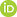Full Text:<5071>

Summary:<1734>

CLC number: TN43

On-line Access: 2014-12-05

Revision Accepted: 2014-06-10

Crosschecked: 2014-11-06

Cited: 0

Clicked: 6146

Citations:  Bibtex RefMan EndNote GB/T7714ORCID:

Ting GUO

http://orcid.org/0000-0002-7853-7479

### -   Go to

Article info.
1. Reference List
 Journal of Zhejiang University SCIENCE C 2014 Vol.15 No.12 P.1200-1210 http://doi.org/10.1631/jzus.C1400091A 37 GHz wide-band programmable divide-by-N frequency divider for millimeter-wave silicon-based phase-locked loop frequency synthesizers

 Author(s):  Ting Guo, Zhi-qun Li, Qin Li, Zhi-gong Wang Affiliation(s):  School of Integrated Circuits, Southeast University, Nanjing 210096, China; more Corresponding email(s):   guotingseu@gmail.com, zhiqunli@seu.edu.cn Key Words:  Wide-band, Divide-by-N, Frequency divider, Dynamic current-mode logic (DCML), Pulse and swallow counters, CMOS Share this article to： More <<< Previous Article|

Ting Guo, Zhi-qun Li, Qin Li, Zhi-gong Wang. A 37 GHz wide-band programmable divide-by-N frequency divider for millimeter-wave silicon-based phase-locked loop frequency synthesizers[J]. Journal of Zhejiang University Science C, 2014, 15(12): 1200-1210.

@article{title="A 37 GHz wide-band programmable divide-by-N frequency divider for millimeter-wave silicon-based phase-locked loop frequency synthesizers",
author="Ting Guo, Zhi-qun Li, Qin Li, Zhi-gong Wang",
journal="Journal of Zhejiang University Science C",
volume="15",
number="12",
pages="1200-1210",
year="2014",
publisher="Zhejiang University Press & Springer",
doi="10.1631/jzus.C1400091"
}

%0 Journal Article
%T A 37 GHz wide-band programmable divide-by-N frequency divider for millimeter-wave silicon-based phase-locked loop frequency synthesizers
%A Ting Guo
%A Zhi-qun Li
%A Qin Li
%A Zhi-gong Wang
%J Journal of Zhejiang University SCIENCE C
%V 15
%N 12
%P 1200-1210
%@ 1869-1951
%D 2014
%I Zhejiang University Press & Springer
%DOI 10.1631/jzus.C1400091

TY - JOUR
T1 - A 37 GHz wide-band programmable divide-by-N frequency divider for millimeter-wave silicon-based phase-locked loop frequency synthesizers
A1 - Ting Guo
A1 - Zhi-qun Li
A1 - Qin Li
A1 - Zhi-gong Wang
J0 - Journal of Zhejiang University Science C
VL - 15
IS - 12
SP - 1200
EP - 1210
%@ 1869-1951
Y1 - 2014
PB - Zhejiang University Press & Springer
ER -
DOI - 10.1631/jzus.C1400091

Abstract:
A 37 GHz wide-band programmable divide-by-N frequency divider (FD) composed of a divide-by-2 divider (acting as the first stage) and a divider with a division ratio range of 273–330 (acting as the second stage) has been designed and fabricated using standard 90 nm CMOS technology. The second stage divider consists of a high-speed divide-by-8/9 dual-modulus prescaler, a pulse counter, and a swallow counter. Both the first stage divider (with high speed) and the divide-by-8/9 prescaler employ dynamic current-mode logic (DCML) structure to improve the operating performance. The first stage divider can work from 2 to 40 GHz and the whole divider covers a wide frequency range from 25 to 37 GHz. The input sensitivity is as low as −20 dBm at 32 GHz and the phase noise at 37 GHz is less than −130 dBc/Hz at an offset of 1 MHz. The whole chip dissipates 17.88 mW at a supply voltage of 1.2 V and occupies an area of only 730 μm ×475 μm.

### Reference

Cheema, H.M., Mahmoudi, R., van Roermund, A., 2010. A 40-GHz phase-locked loop front-end for 60-GHz transceivers in 65nm CMOS. IEEE Asia Pacific Conf. on Circuits and Systems, p.967-970.Chen, H.K., Wang, T., Lu, S.S., 2011. A millimeter-wave CMOS triple-band phase-locked loop with a multimode LC-based ILFD. IEEE Trans. Microw. Theory Tech., 59(5):1327-1338.Ding, Y., Kenneth, K.O., 2007. A 21 GHz 8-modulus prescaler and a 20-GHz phase-locked loop fabricated in 130-nm CMOS. IEEE J. Solid-State Circ., 42(6):1240-1249.Eschenko, E., Candidate, M.S., Entesari, K., 2007. A low noise 13 GHz power efficient 16/17 prescaler with rail to rail output amplitude. Proc. 50th Midwest Symp. on Circuits and Systems, p.427-430.Gai, X.L., Chartier, S., Trasser, A., et al., 2011. A 35 GHz dual-loop PLL with low phase noise and fast lock for millimeter wave applications. IEEE MTT-S Int. Microwave Symp. Digest, p.1-4.Gao, Z.Q., Xu, Y.X., Sun, P., et al., 2010. A programmable high-speed pulse swallow divide-by-N frequency divider for PLL frequency synthesizer. Int. Conf. on Computer Application and System Modeling, p.V6.315-V6.318.Guo, T., Li, Z.Q., Li, Q., et al., 2012. A 7-27 GHz DSCL divide-by-2 frequency divider. J. Semicond., 33(10):105006.Hammad, M.C., Mahmoudi, R., van Zeijl, P.T.M., et al., 2010. A 40-GHz phase-locked loop for 60-GHz sliding-IF transceivers in 65nm CMOS. IEEE Asian Solid State Circuits Conf., p.1-4.Jau, T.S., Yang, W.B., Lo, Y.L., 2006. A new dynamic floating input D flip-flop (DFIDFF) for high speed and ultra low voltage divided-by 4/5 prescaler. Proc. 13th IEEE Int. Conf. on Electronics, Circuits and Systems, p.902-905.Luo, T., Chen, Y., 2008. A 0.8-mW 55-GHz dual-injection-locked CMOS frequency divider. IEEE Trans. Microw. Theory Tech., 56(3):620-625.Murphy, D., Gu, Q.J., Wu, Y., et al., 2011. A low phase noise, wideband and compact CMOS PLL for use in a heterodyne 802.15.3c transceiver. IEEE J. Solid-State Circ., 46(7):1606-1617.Pellerano, S., Mukhopadhyay, R., Ravi, A., et al., 2008. A 39.1-to-41.6GHz ΔΣ fractional-N frequency synthesizer in 90nm CMOS. IEEE Int. Solid-State Circuits Conf., Digest of Technical Papers, p.484-630.Reyes, N., Zorzi, P., Jarufe, C., et al., 2010. Construction of a heterodyne receiver for band 1 of ALMA. Proc. 21st Int. Symp. on Space Terahertz Technology, p.366-367.

Sheng, N.H., Pierson, R.L., Wang, K.C., et al., 1991. A high-speed multimodulus HBT prescaler for frequency synthesizer applications. IEEE J. Solid-State Circ., 26(10):1362-1367.Usama, M., Kwasniewski, T.A., 2006. A 40-GHz frequency divider in 90-nm CMOS technology. IEEE North-East Workshop on Circuits and Systems, p.41-43.Vaucher, C.S., Ferencic, I., Locher, M., et al., 2000. A family of low-power truly modular programmable dividers in standard 0.35-/spl mu/m CMOS technology. IEEE J. Solid-State Circ., 35(7):1039-1045.Wang, K.P., Ma, K.X., Yeo, K.S., 2012. Low-power high-speed dual-modulus prescaler for Gb/s applications. IEEE Asia Pacific Conf. on Circuits and Systems, p.256-259.Wong, K.L.J., Rylyakov, A., Yang, C.K.K., 2005. A broadband 44-GHz frequency divider in 90-nm CMOS. IEEE Compound Semiconductor Integrated Circuit Symp., p.196-199.Yang, C.Y., Dehng, G.K., Liu, S.I., 1997. High-speed divide-by-4/5 counter for a dual-modulus prescaler. Electron. Lett., 33(20):1691-1692.